### 1 Introduction to Electromagnetics

Electromagnetic fields are caused by electric charges at rest and in motion. Positive and negative electric charges are sources of the electric fields and moving electric charges yielding a current is the source of magnetic fields. Time-varying electric and magnetic fields are coupled in an electromagnetic field radiating from the source.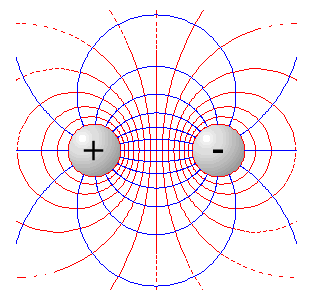Figure 1 Positive and negative electric charges are sources of an electric field

Electromagnetic fields are divided into four different quantities:

• the magnetic flux density B with the unit T (Tesla or volt-second per square meter)
• the magnetic field intensity H with the unit A/m (Ampere per meter)
• the electric field intensity E with the unit V/m (Volt per meter)
• the electric flux density D with the unit C/m2 (Coulomb per square meter)

A time-varying E and D will give rise to B and H, and vice versa where the relation depends on the properties of the medium. Far enough from the source the magnetic field, H, will be perpendicular to the electric field, E, and both are normal to the direction of propagation, as shown in the following figure: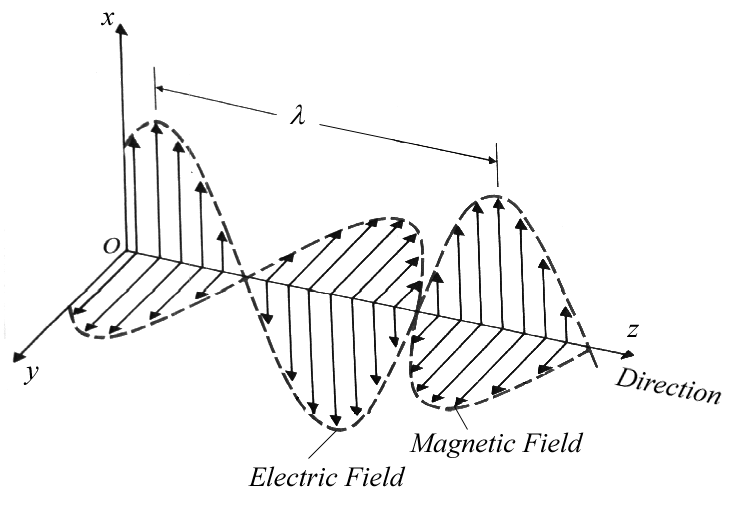Figure 2 A time-varying electric field, E, will give rise to a perpendicular magnetic field, H, and vice versa. Far enough from the source it will become a uniform plane wave and the ratio between E and H will be the intrinsic impedance of the medium.

Far enough from the source, the wave-front, which will become almost spherical, can be seen as an almost plane front if the sphere is large enough. Then we have a uniform plane wave where the ratio between the electric field and the magnetic field, called the wave impedance: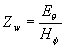Equation 1

is a constant named the intrinsic impedance of the medium, h . The electromagnetic theory is also based on three universal constants:

• the velocity of an electromagnetic wave in free space c (the speed of light » 3.108 m/s)
• the permittivity of free space e 0 (» 8.854.10-12 F/m)
• the permeability of free space m 0 (= 4p .10-7 H/m)

These constants are related by :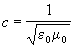Equation 2

The permittivity is a proportionality constant between the electric flux density D and the electric field intensity E, in free space as: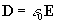Equation 3

and the permeability is the proportionality constant between the magnetic flux density B and the magnetic field intensity H, in free space as: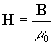Equation 4

From these constants, the intrinsic impedance of free space can be calculated as :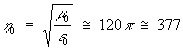[W ] Equation 5

Next: Maxwell's Equations

EMC of Telecommunication Lines
A Master Thesis from the Fieldbusters © 1997
Joachim Johansson and Urban Lundgren